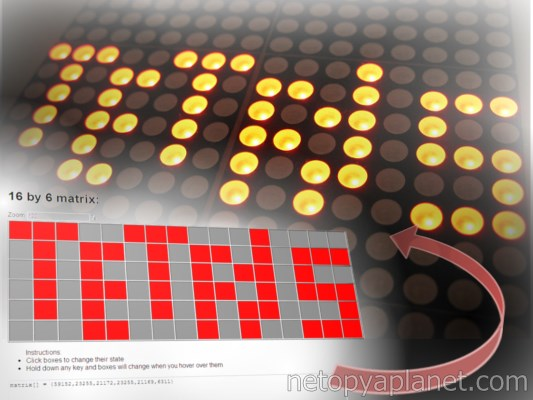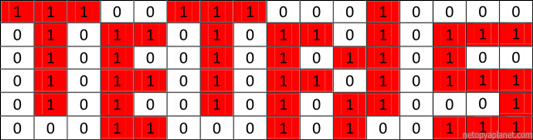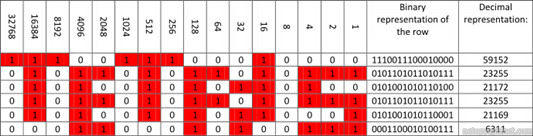Social:

# LED Matrices with Arduino, Netopya's Way

## By Netopya on Fri Jan 17th 2014 3:57 PMOne of the great things I learned working on my previous project, the AEMD Alpha, was using the Arduino to control an LED matrix. The Adafruit libraries for the device took care of the multiplexing and other complexities and all that was left was controlling the individual LEDs from their x and y coordinates. But this led to the next challenge of how exactly to get images and graphics on the Arduino. First I’ll describe a logic solution, but I’ll show how this method falls apart. The alternative solution requires arrays of bytes (or integers) which is where my previous post introducing Bytety comes in. This little web app that I created presents a mock matrix that you can play around with to create your own graphics, and the program generates the necessary array for your Arduino code. Continue reading on to learn my easy solution to display images on a LED matrix with an Arduino all while improving the memory footprint of your program.

Notes: A lot of what I will be discussing is addressed on this site here, which is one of the places where I learnt how to do this. In my post, I will be using as an example the LED matrix that I used in the AEMD project, the Adafruit 16x24 matrix (#555).

The way that I originally thought of addressing this challenge was to make a 2-dimensional arrays of Booleans with one variable for each pixel, as illustrated in this table to show the word TETRIS:Fig. 1 - Table representing the pattern of pixels required to display the word tetris

And here is how I would code this word on 6 rows of a matrix with the supporting code to display it on the LEDs. Note how the array tetris corresponds to the rows and columns of the table above.

The problem with this method is that it isn’t exactly elegant, and it’s huge code wise and more importantly memory wise. Each Boolean takes one byte of memory which makes the example use 96 bytes. This may not sound like much and isn’t too bad if this is your only graphic in the program, but if you need more images and text, this method adds up really fast making it really easy to run out of memory on small platforms like the Arduino. We can do better! The alternative is to store each row in as a binary number, combining each piece of data into one integer. In the table below, each row is packed into a binary value as shown in the last column. A binary number can be written using the 0b prefix in Arduino code, or you can calculate the 10 based decimal representation by, using the example below, summing up all the products of the columns with the value in the top row, for the respective row.Fig. 3 - Representing rows of pixels with as a decimal nummber

Here are the two possible alternatives to the tetris array, the first one in binary and the second one in decimal, both of which are now 1-dimensional arrays. I like using the decimal representation since it visually takes up less space. Therefore, note that I must use unsigned integers. In the Arduino’s language, integers are 16-bits which is convenient since this is the number of columns on the LED matrix. But by default the last bit on integers determine the signing of the number and not the actual absolute value of the number, so I must specify the unsigned nature of the variables to allow the use of all 16-bits. Below I show the changed `tetris[]` array using the decimal representation of the rows.

As you can tell by my explanation so far, this process to create your array is somewhat complicated, which is why I created the Bytety app to simplify to process. First enter in the dimensions of your LED matrix, and then click on the boxes to toggle the state of the LED they represent. You can also hold down any key to then hover over the boxes to change multiple LEDs at once. The array of integers as decimal representations of the binary values of the rows is generated for you!

Now I will explain how to display this on an LED Matrix, as I did for the AEMD project. Below I give below an example of how to implement this in your code to show the word TETRIS.

As you can see, I’ve changed the code a lot. First of all, I use the Arduino’s PROGMEM features to store and retrieve the variable in the Arduino’s flash memory to further improve the memory efficiency of the program (See the Arduino Reference). I write `PROGMEM prog_uint16_t` in front of the variable to declare it and then use `pgm_read_word(&(VARIABLE_NAME)) ` to read the variable. If your graphic uses less than 9 columns, then you should probably use bytes in the place of integers (and change the PROGMEM codes accordingly) so save on memory. Now if we look at the value of tetris; this is where I store the byte array (as I explained I used integers instead) that contains all the information for the LED states with each item in the array corresponding to a row of LEDs.

To decode this information and display it on the matrix, I use the code in the `loop()`. Let’s break it down step by step. First is the two nested for loops to run through the x and y coordinates of all the LEDs we will be displaying. Secondly is the matrix.drawPixel(y,x,value) function which comes from the Adafruit library for the display and allows us to set the state of individual pixels. Note how I inverted the x and y values since the display is meant to be used in landscape while I’m using it in portrait. Next is a function I created called toeightbit(). The Adafruit library expects and 8-bit variable for the state of the LED like a Boolean. But I’m using 16-bit integers and the output of the code to determine the state of the individual pixels is also an integer, so I must use this function to convert it to a Boolean. Next I use the `pgm_read_word(&(VARIABLE_NAME)) ` function to read the variable the tetris variable where I stored all the information, but I use y as the index to extract the row of pixels for the graphic.

Now that we have the row, we must check each column and see whether we want that pixel to be on or off. ` 1u << x` is a bit shift operation that produces a binary number with a one in the column that we wish to check. Comparing this number using a bitwise And (`&`) to the value of the row from ` tetris[y]` results in 0 if there is not a 1 value in the position that we are checking in ` tetris[y]`, or the result of the bitwise operation if there is a 1 value in that position (which is some number that is not zero, infact it’s ` 1u << x`). So moving back through my explanation, this value gets set to the toeightbit() function which converts it to a Boolean which then sets the value of matrix.drawPixel(y,x,value), either setting the pixel on or off.>

Having a general understanding of the concepts in this tutorial such as bitwise operations can be very useful and I would recommend it for your own implementations of these ideas. Understanding the logic behind byte arrays and their use is very important, even though the Bytety app I created can make these byte arrays for you; it should serve as a complement tool. I hope you’ve learnt a lot about making more efficient and smaller programs and make much use of Bytety!